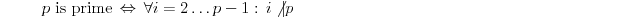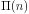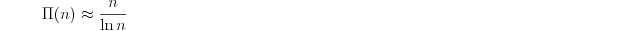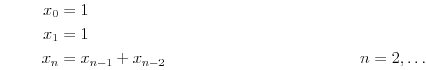## 2 Mathematical formulas

##### Demonstratie 2.1

This section shows the use of mathematical formulas.

### 2.1 Prime numbers

We start with a definition. There is no extra element for it, we use the remark element with class definition:

#### Slide 2.1 Defintion of prime numbers

##### Definitie 2.1 Prime number

A positive integer is prime, if it has exactly two dividors:For a theorem, we use a remark element with class theorem. We put it also on a slide:

#### Slide 2.2 Amount of Prime Numbers

##### Stelling 2.2 Prime number theorem

The amount of prime numbersup to a numberis approximately:For inline formula use the element m (see slide 2.2). The element eq is used for block formulas. With class align multiline formulas are possible:The CDATA declaration is necessary to protect the & signs. If you add the id attribute, you can set a reference to this formula 2.2.